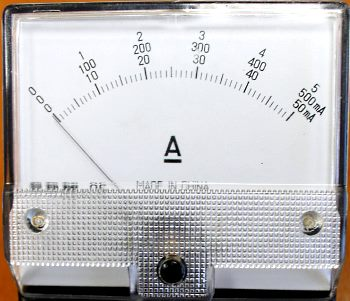calculator.org

# property>electric current

## What is Electric Current?Electric current is the rate of flow of electric charge through a conductor in an electric circuit. It is measured in coulombs per second (C/s) or amperes (the SI unit for electric current). A charge flows when an electromotive force is applied, given by potential difference (or voltage). The charge always flows from high electrical potential to low potential. Electric current can be simply called “current”. The electric charge is carried by mobile electrons in a conductor, or ions in an electrolyte.

Electric current is measured by an ammeter. By definition electric current is measured as I=Q/t, but the definition is still true if you calculate current by I=V/R (Ohm’s law). Here ‘Q’ is charge, ‘I’ is electric current, ‘t’ is time, ‘V’ is Voltage and ‘R’ is Resistance. In Ohm’s law the potential difference (V) across an ideal resistor is directly proportional to the current flowing in the resistor.

Electric current can flow through various media. A solid conductive metal, for example, contains free electrons. Even when there is no external electric field or potential applied these electrons move randomly due to thermal energy but there is zero net current within the metal. When an electric potential is applied these free electrons move in the direction of the electromotive force and we call this electric current. In metals the positive charge carriers are the atoms which are motionless. Only the electrons, which are negative charge carriers, actually flow; so the direction of motion of the electrons in the metal is actually in the opposite direction to the “conventional” electric current. In the case of a battery the conventional current flows from the positive to the negative terminal, but in reality the electrons are moving from the negative to the positive terminal.

Electric current is also produced naturally by various phenomena including lightning, electric eels, solar wind and the polar auroras. The nervous system in humans and animals also uses a form of electric current based on the exchange of ions.

The main electricity supply consists of electric current flowing through copper wires over great distances in overhead or, increasingly, underground power cables. These transfer electrical energy through a distribution grid from the generators in the power stations. Electrical current is very useful but it is also dangerous and can kill you if you mishandle it. A current of only a few milliamps can be fatal and passes easily through the body given a sufficiently high voltage. A very small current will cause a tingling feeling, but if it is too large it can cause severe burns and if the current passes through the heart it will interfere with the electrical impulses which control it, leading to death. Ultimately it is not the voltage that indicates the level of danger, but the resulting current that flows through the body.

Bookmark this page in your browser using Ctrl and d or using one of these services: (opens in new window)